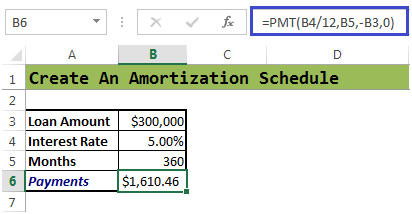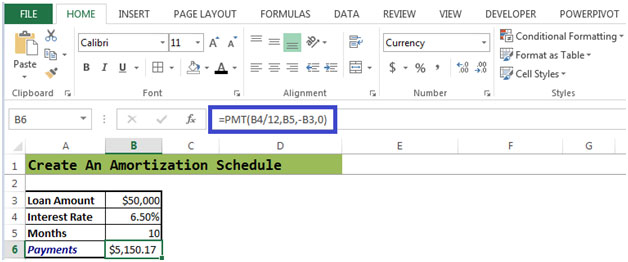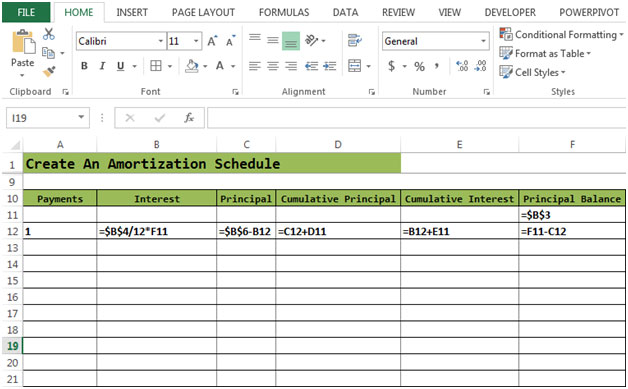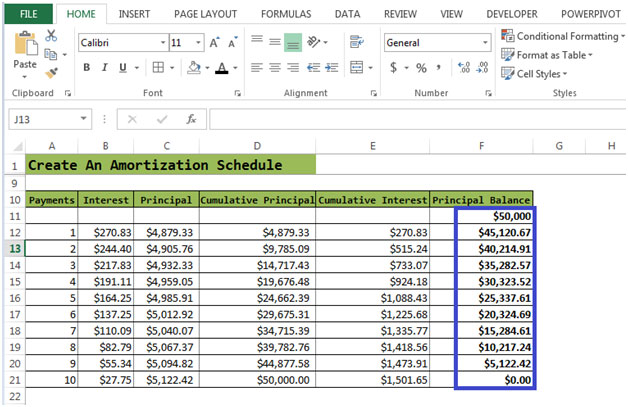# Loan Amortization Schedule

In this article we will learn about how to calculate the loan amortization schedule in Excel. To calculate loan payment we will use the “RATE”, “NPER”, “PV”, “PMT”, “PPMT” and “IPMT” formulae. All these formulae will help to create the amortization table in Excel.

PMT: Returns the regular monthly payment on the loan (principal + interest) when the interest for each of the monthly payments is constant. Calculates the payment for a loan based on constant payments and a constant interest rate.

Syntax of “PMT” function: =PMT (Rate, Nper, -Loan Amount)
RATE: Returns the percentage of interest on the loan, when the number of payments is constant. Rate is calculated by iteration can have zero or more solutions.

Syntax of “NPER” function: =NPER (Rate, Pmt, -Loan Amount)
PV: The present value, the total amount that a series of future payments is worth now. Returns the current value for a series of payment with a constant interest rate.

Syntax of “PV” function: =PV (Rate, Nper, Pmt)
PPMT: Returns the amount on the principal for a given period for a loan based on periodic, constant payments and a constant interest rate. And also returns the sum of the principal within the monthly payment (the monthly payment is comprised of the principal + interest).

Syntax of “PMT” function: =PPMT (Rate, Which Period, Nper, -Loan Amount)
IPMT: Returns the interest payment for a given period for a loan based on periodic, constant payments and a constant interest rate. And also Returns the amount of the interest within the monthly payment (the monthly payment is comprised of the principal + interest).

Syntax of “IPMT” function: =IPMT (Rate, Which Period, Nper, -Loan Amount)
Let’s take an example:

• The Bank has approved \$300,000as the loan amount
• Interest Rate is 5% floating rate
• Number of Months 360 (12 x 30)
• We need to calculate the payments to be made every month
• We need to calculate themoney we are paying towards interest each month

Determining a monthly payment

• If there was no interest rate, monthly payment would be (\$300,000 / 360 = \$833.33)
• We can calculate the the monthly payment by using the PMT function

Syntax =PMT(rate,nper,pv,fv,type)

Let’s have a quick look at the arguments of this function.

• Cell B4 has the Interest Rate which is divided by 12, as it is the annual rate which is
calculated monthly.
• Cell B5 has “nper” which is calculated by multiplying Number of years the loan is
required by 12 months. 10 is the number of months as shown in above example.
• Cell B3 has Present Value.
• Type is for payment at the end of the period, for which we will enter‘0’ or we can
also omit it. For payments made at the beginning of the period we will enter 1.
• Cell B6=PMT(B4/12,B5,-B3,0)
• The monthly payment is \$ 1,610.46The PMT function is used to calculate the periodic payment for a standard amortizing loan.
Lets take another example -

See Figure 2 below:

Figure 2The monthly payment is \$ 5,150.17 for a loan amount of \$50,000 and an interest rate of 6.50% for a period of 10 months.

Figure 2a

This figure shows the formulae which have been entered in the required cells.

In Figure 2a, Principle Balance in cell F11=Loan Amount
Principle Balance in cell F12=F11-C12(Interest)Let’s calculate the Interest, Principal, Cumulative Principal & Cumulative Interest

• Interest is calculated in cell B12=\$B\$4/12*F11 as per figure2b.
• Formula for Principal in cell C12= Interest in cell B12 is subtracted from
Payments in cell \$B\$6.
• Formula for Cumulative Principal in cell D12=C12+D11
• Formula for Cumulative Interest in cell E12 =B12+E11

After entering all the formulae:

• You need to select cell B12 to F12
• Then with your mouse, click and hold the square dot on the lower-right corner of the
selection
• Then drag to extend the selection to the number of payments till Principal Balance
becomes zero.

Figure 2bThe process of interest calculation based on the remaining balance continues until the mortgage is paid off. So each month the amount of interest decreases and amount to pay-off the loan increases. After 10 payments, the mortgage is fully paid off.

## Users are saying about us...

1.what is the formula used to calculate a mortgage payment that includes tax, insurance and any other payment included in my monthly payment?

2.Is it possible to create an amortization spreadsheet that will update (estimate) the associated totals (i.e., prn bal,int paid, int due,etc..) as the payments are entered each month?

3.What would be the written formula to recalculate the number of payments in months if the interest rate was lowered but the payment stayed the same.

4.If I know principal, interest, and # years, how do I calculate total interest paid over the life of the loan considering monthly payments?

5.How do I make a amortization sheet for a personal loan to another party.

6.“Hi Scott,

Don’t know if you still need this answer, but anyway:

If thee are other elements that principle and interest in the periodic payments, then you will need to eliminate them from the payment before applying it to your loan calculation.

Just do that calculation separately in your model before getting into the loan calculation zone.

Alan.”

7.“Hi Charles,

Yes you can do this.

Just set up your repayment schedule to refer to the brought forward balance each period (rather than calculate the brought forward balance by reference to the opening scenario).

If you also include a cell for the ‘current’ interest rate and that is entered each period, you can also generalise for a floating rate calculation, and if you like, forecast foreward what the rates will be.

That approach is also self-correcting if the borrower misses payments or short-pays a period, since it re-claculates based on actuals, not the original theoretical repayment schedule.

You might also want to set up the calculation with extra periods past the original scheduled closure date. That way you can cope with someone who misses or short-pays and then continues with the original repayments, thus extending the period of the loan.

Alan.”

8.“Hi Des,

It sounds like all you need is to use the NPER function which, “”returns the number of periods for an investment based on periodic, constant payments and a constant interest rate.””

If the rate changes, just re-calculate using NPER or set it up so that each period is re-calculated based on the previous actuals in preceding periods (as for the answer above).

Alan.”

9.“Jeff,

Sorry!

Alan”

10.“Hi Shannon,

Using the following example:

Interest rate: 10% pa
Periods: 60 (5 years, monthly)
Initial loan: \$10,000
Final balance = \$Nil
Interest charged at end of period

To get the interest payable in any one given period, we can use the IPMT function. For example, the fifth month interest is:

IPMT((110%^(1/2))-1,5,60,10000,0,0)

To get the total interest paid, we simply sum that function for all periods (1 through 60 inclusive), ut entering the following as an ARRAY FORMULA:

{=SUM(IPMT((110%^(1/2))-1,ROW(1:60),60,10000,0,0))}

Note that this must be entered with Shift-Ctrl-Enter to get the braces around the formula. Check Excel’s help for more about array formulae.

Alan.”

11.“Hi Bobby,

Isn’t that exactly what the examples on this pge show you?

If not, please post back with a more detailed query and I’m sure we can help.

Thanks,

Alan.”

12.what is the formula used to calculate a mortgage payment that includes tax, insurance and any other payment included in my monthly payment?

13.Is it possible to create an amortization spreadsheet that will update (estimate) the associated totals (i.e., prn bal,int paid, int due,etc..) as the payments are entered each month?

14.What would be the written formula to recalculate the number of payments in months if the interest rate was lowered but the payment stayed the same.

15.If I know principal, interest, and # years, how do I calculate total interest paid over the life of the loan considering monthly payments?

16.How do I make a amortization sheet for a personal loan to another party.

17.“Hi Scott,

Don’t know if you still need this answer, but anyway:

If thee are other elements that principle and interest in the periodic payments, then you will need to eliminate them from the payment before applying it to your loan calculation.

Just do that calculation separately in your model before getting into the loan calculation zone.

Alan.”

18.“Hi Charles,

Yes you can do this.

Just set up your repayment schedule to refer to the brought forward balance each period (rather than calculate the brought forward balance by reference to the opening scenario).

If you also include a cell for the ‘current’ interest rate and that is entered each period, you can also generalise for a floating rate calculation, and if you like, forecast foreward what the rates will be.

That approach is also self-correcting if the borrower misses payments or short-pays a period, since it re-claculates based on actuals, not the original theoretical repayment schedule.

You might also want to set up the calculation with extra periods past the original scheduled closure date. That way you can cope with someone who misses or short-pays and then continues with the original repayments, thus extending the period of the loan.

Alan.”

19.“Hi Des,

It sounds like all you need is to use the NPER function which, “”returns the number of periods for an investment based on periodic, constant payments and a constant interest rate.””

If the rate changes, just re-calculate using NPER or set it up so that each period is re-calculated based on the previous actuals in preceding periods (as for the answer above).

Alan.
Re: Above
Alan Posted on: 31-12-1969

Jeff,

Sorry!

Alan”

20.“Hi Shannon,

Using the following example:

Interest rate: 10% pa
Periods: 60 (5 years, monthly)
Initial loan: \$10,000
Final balance = \$Nil
Interest charged at end of period

To get the interest payable in any one given period, we can use the IPMT function. For example, the fifth month interest is:

IPMT((110%^(1/2))-1,5,60,10000,0,0)

To get the total interest paid, we simply sum that function for all periods (1 through 60 inclusive), ut entering the following as an ARRAY FORMULA:

{=SUM(IPMT((110%^(1/2))-1,ROW(1:60),60,10000,0,0))}

Note that this must be entered with Shift-Ctrl-Enter to get the braces around the formula. Check Excel’s help for more about array formulae.

Alan.”

21.“Hi Bobby,

Isn’t that exactly what the examples on this pge show you?

If not, please post back with a more detailed query and I’m sure we can help.

Thanks,

Alan.”

22.Is it possible using excel amortization sheet to create the coupon booklet using same existing entries in cell? I can not find anything that does and bring over dates.

23.Can a coupon book be made using same amortization schedule?

Terms and Conditions of use

The applications/code on this site are distributed as is and without warranties or liability. In no event shall the owner of the copyrights, or the authors of the applications/code be liable for any loss of profit, any problems or any damage resulting from the use or evaluation of the applications/code.